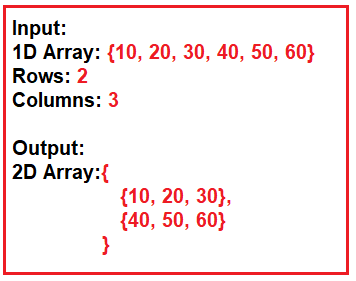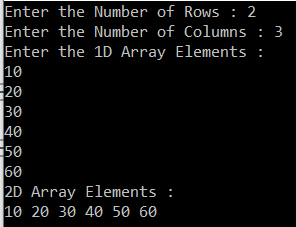# How to convert a one-dimensional array to a two-dimensional array in C#

## How to convert a one-dimensional array to a two-dimensional array in C#

In this article, I am going to discuss how to convert a one-dimensional array to a two-dimensional array in C# with an example. Please read our previous article where we discussed how to convert a two-dimensional array to one-dimensional array in C# with some examples.

##### Program Description:

Here, the user will input a one-dimensional array along with the number of rows and columns for the two-dimensional array. Then we need to convert the one-dimensional array to a two-dimensional array based on the given rows and columns. For a better understanding of what we want to do, please have a look at the following diagram.Note: The number of elements in the array should be the multiplication of the number of rows and number of columns.

##### Creating a 2d Array from 1D Array in C#:

In the following program, first, we take the number of rows and columns for the 2d array from the user. Then we create and populate the 1d array with the required data. Based on the number of rows and columns we created the 2d array and using two loops we populate the 2d array with data coming from the 1d array.

```using System;
namespace LogicalPrograms
{
class Program
{
static void Main(string[] args)
{
Console.Write("Enter the Number of Rows : ");

Console.Write("Enter the Number of Columns : ");

//Creating a 1d Array
Console.WriteLine("Enter the 1D Array Elements : ");
int[] oneDimensionalArray = new int[Rows * Columns];
for(int i = 0; i<oneDimensionalArray.Length; i++)
{
}

//Creating 2d Array
int index = 0;
int[,] twoDimensionalArray = new int[Rows, Columns];

for (int x = 0; x < Rows; x++)
{
for (int y = 0; y < Columns; y++)
{
twoDimensionalArray[x, y] = oneDimensionalArray[index];
index++;
}
}

//Printing the 2D array elements
Console.WriteLine("2D Array Elements : ");
foreach (int item in twoDimensionalArray)
{
Console.Write(item + " ");
}